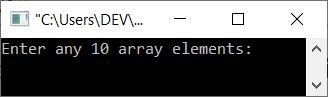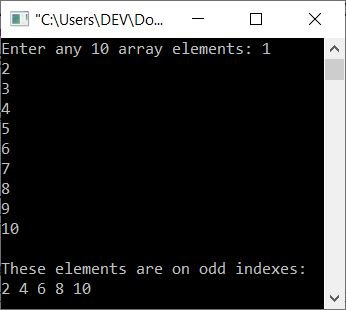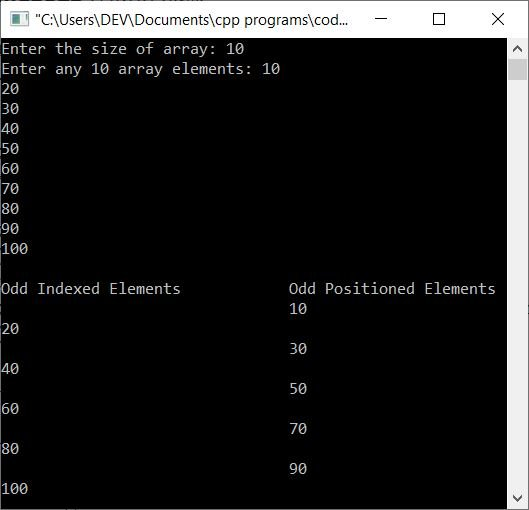# C++ Program to Print Elements Available in Odd Positions in an Array

This article provides some programs in C++ that find and print all elements available in odd positions. This article covers mainly these two programs:

Note: Index starts with 0, therefore the first element is the element that is available at the 0th index of the array.

For example, if an array arr[] holds 1, 2, 3, 4, 5, and 6 as its six elements, then elements at odd indexes are elements at arr, arr, and arr, which are 2, 4, and 6.

## Print Elements on Odd Indexes

The question is: write a C++ program that receives any 10 numbers for the array and prints all numbers available on odd indexes. The program given below is the answer to this question:

```#include<iostream>

using namespace std;
int main()
{
int arr, i;
cout<<"Enter any 10 array elements: ";
for(i=0; i<10; i++)
cin>>arr[i];
cout<<"\nThese elements are on odd indexes:\n";
for(i=0; i<10; i++)
{
if(i%2!=0)
cout<<arr[i]<<" ";
}
cout<<endl;
return 0;
}```

The initial output produced by the above C++ program on printing odd-indexed elements in an array is shown in the snapshot given below:Now supply any 10 elements, say 1, 2, 3, 4, 5, 6, 7, 8, 9, and then press the ENTER key to print all numbers available on odd indexes like shown in the snapshot given below:## Print Elements in Odd Positions

To create this program, just replace the following code from the above program:

`if(i%2!=0)`

with the code given below:

`if((i+1)%2!=0)`

Here is the complete version of the program:

```#include<iostream>

using namespace std;
int main()
{
int arr, i;
cout<<"Enter any 10 array elements: ";
for(i=0; i<10; i++)
cin>>arr[i];
cout<<"\nThese elements are on odd indexes:\n";
for(i=0; i<10; i++)
{
if((i+1)%2!=0)
cout<<arr[i]<<" ";
}
cout<<endl;
return 0;
}```

Here is another sample run with the same user input as the previous program:#### Allow the user to define the size of the array too

This program combines the previous two programs, as well as allowing the user to specify the size of the array:

```#include<iostream>

using namespace std;
int main()
{
int i, n;
cout<<"Enter the size of array: ";
cin>>n;
int arr[n];
cout<<"Enter any "<<n<<" array elements: ";
for(i=0; i<n; i++)
cin>>arr[i];
cout<<"\nOdd Indexed Elements\t\tOdd Positioned Elements\n";
for(i=0; i<n; i++)
{
if(i%2!=0)
cout<<arr[i]<<endl;
if((i+1)%2!=0)
cout<<"\t\t\t\t"<<arr[i]<<endl;
}
cout<<endl;
return 0;
}```

Here is its sample run with user input of 10 as size and 10, 20, 30, 40, 50, 60, 70, 80, 90, and 100 as ten elements:C++ Quiz

« Previous Program Next Program »